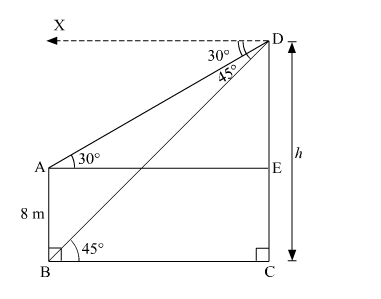# The angles of depression of the top and bottom of a 8 m tall building from the top of a tower are 30° and 45°`
Question:

The angles of depression of the top and bottom of a 8 m tall building from the top of a tower are 30° and 45° respectively. Find the height of the tower and the distance between the tower and the building.

Solution:

Let AB be the building and CD be the tower.

Draw AE ⊥ CD.Suppose the height of the tower be h m.

Here, AB = 8 m

∠DAE = ∠ADX = 30º        (Alternate angles)

∠DBC = ∠BDX = 45º        (Alternate angles)

CE = AB = 8 m

∴ DE = CD − CE = (h − 8) m

Distance between the building and tower = BC

In right ∆BCD,

$\tan 45^{\circ}=\frac{\mathrm{CD}}{\mathrm{BC}}$

$\Rightarrow 1=\frac{h}{\mathrm{BC}}$

$\Rightarrow \mathrm{BC}=h \mathrm{~m}$

In right ∆AED,

$\tan 30^{\circ}=\frac{\mathrm{DE}}{\mathrm{AE}}$

$\Rightarrow \frac{1}{\sqrt{3}}=\frac{h-8}{h} \quad(\mathrm{AE}=\mathrm{BC})$

$\Rightarrow \sqrt{3} h-8 \sqrt{3}=h$

$\Rightarrow \sqrt{3} h-h=8 \sqrt{3}$

$\Rightarrow(\sqrt{3}-1) h=8 \sqrt{3}$

$\Rightarrow h=\frac{8 \sqrt{3}}{\sqrt{3}-1}$

$\Rightarrow h=\frac{8 \sqrt{3}(\sqrt{3}+1)}{(\sqrt{3}-1) \times(\sqrt{3}+1)}$

$\Rightarrow h=\frac{8 \sqrt{3}(\sqrt{3}+1)}{2} \quad\left[(a-b)(a+b)=a^{2}-b^{2}\right]$

$\Rightarrow h=4 \sqrt{3}(\sqrt{3}+1)$

$\Rightarrow h=(12+4 \sqrt{3}) \mathrm{m}$

$\therefore$ Height of the tower $=(12+4 \sqrt{3}) \mathrm{m}$

Also,

Distance between the tower and building $=\mathrm{BC}=(12+4 \sqrt{3}) \mathrm{m}$        (BC = h m)

Thus, the the height of the tower is $(12+4 \sqrt{3}) \mathrm{m}$ and the distance between the tower and the building is $(12+4 \sqrt{3}) \mathrm{m}$.